##### Search### SECTION I (50 Marks)

Answer all questions in this section
1.

Without using Mathematical tables or calculators, evaluate,3 marks

2.

A Kenyan business lady received US Dollars 100,000. The money was converted into Kenya shillings in a bank which buys and sells foreign currencies as follows.

1 US Dollar 100.10 100.22

1 Sterling pound 121.93 122.27
a) Calculate the amount of money in Kenya shillings, the lady received.

b) The lady exchanged the Kenya shillings calculated in (a) above for sterling pounds to buy a car from Britain. Calculate the cost of the car to the nearest sterling pounds.

3 marks

3.

Solve the simultaneous equations ;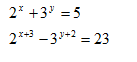4 marks

4.

Simplify the expression completely;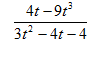3 marks

5.

Vector P passes through the points (10, 20) and (6, 10) while vector q passes through the points (x, 12) and (-10, -8). If

p and q are parallel, find the value of x

2 marks

6.

On a map with scale 1: 50,000, a square piece of land has an area of 64 ha. Determine its dimensions in cm on the map

3 marks

7.

. Find y if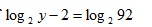3 marks

8.

Calculate the area of the trapezium below.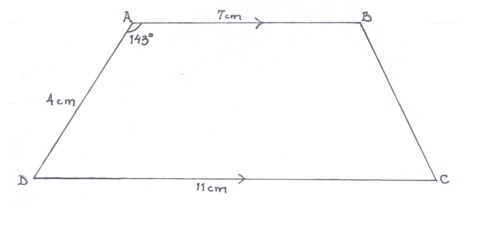3 marks

9.

A student walks directly from point A towards the foot of a building 200M away. After covering 140m, he observes that the angle of elevation of the top of the building is 450. Determine the angle of elevation of the top of the building from A.

3 marks

10.

A fruiter bought 144 pineapples at Ksh. 100 for every six pineapples. She sold some of them at Ksh. 72 for every three and the rest Ksh. 60 for every two. If she made a 65% profit, calculate the number of pineapples sold at Ksh. 72 for every three.

3 marks

11.

The radii of two similar tanks are 20cm and 4M respectively. The volume of the smaller tank is 35.2 litres. Calculate the capacity of the large tank, giving your answer in standard form.

3 marks

12.

. a) Using a ruler and a pair of compasses only, construct triangle ABC such that AB =5cm, AC= 11.2cm and <ABC = 1350

b) i) Measure BC.

ii) Drop a perpendicular from C to meet AB produced at D.

4 marks

13.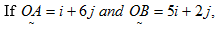determine the length AB to 2 d.p.

2 marks

14.

The size of each interior angle of a regular polygon is four times the size of the exterior angle. Find the number of sides of the polygon.

3 marks

15.

a) Find the range of values of x which satisfies the following inequalities simultaneously.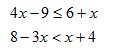b) Show the solution in (a) above on a number line

3 marks

16.

Use logarithm to evaluate,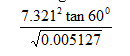3 marks

### SECTION II (50 Marks)

Answer only five questions in this section
17.

A solid consists of a cone and a hemisphere. The common diameter of the cone and hemisphere is 12cm and the slanting height of the cone is 10cm.

a) Calculate correct to 2d.p

i) The surface area of the solid.

ii) The volume of the solid.

b) If the density of the material used to make the solid is 1.3g/cm3; calculate its mass in kg.

10 marks

18.

The figure below shows the floor of a hall. A part of this floor is in the shape of a rectangle of length 20m and width 16m and the rest is a segment of a circle centre O of radius 12m.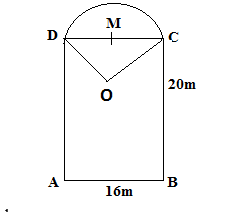Use the figure to find:

a) The size of angle COD.

b) The area of figure.

c) The cost of carpeting the floor with tiles if carpeting costs sh 76 per 900cm2

10 marks

19.

A bus left Bungoma and travelled towards Nairobi at an average speed of 60km/h. After 2 hours, a car left Bungoma and travelled along the same road at an average speed of 90km/h. If the distance between Bungoma and Nairobi is 400km, determine

a) i) the distance of the bus from Nairobi when the car took off.

ii) the distance the car travelled to catch up with the bus.

b) Immediately the car caught up with the bus, the car stopped for 20 minutes. Find the new average speed at which the car travelled in order to reach Nairobi at the same time as the bus.

10 marks

20.

The vertices of a triangle ABC are A(2,0), B(4,2) and C(0, 3). The vertices of its image under a rotation are A1(1, -3), B1(3,-5) and C1(4,-1).

a) i) On the grid below draw triangle ABC and its image A1B1C1ii) a) Determine the centre and angle of rotation.

b) On the same grid draw triangle A11B11C11, the image of A1B1C1 under a reflection in the line y = x.

c) On the same grid draw triangle A111 B111C111, the image of A11B11C11 under enlargement scale factor -1 centre (2,-1). State the co-ordinates of A111B111C111.

10 marks

21.

Two aeroplanes S and T, leaves Bungoma airstrip at the same time, S flies on a bearing of 0600 at 750km/h while T flies on a bearing of 2100 at 900km/h a) Using suitable scale, draw a diagram to show the positions the aeroplanes after two hours

b) Use your diagram to determine

i) The actual distance between the two planes.

ii) The bearing of T from S.

iii) Determine the shortest distance from Bungoma to route ST.

10 marks

22.

. The figure below shows two circles each of radius 7cm, centres at X and Y.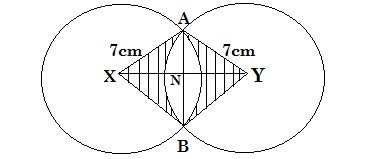Given that angle AXN = 300. Find

a) The length of chord AB.

b) The area of triangle AXB

c) The area of the shaded region.

10 marks

23.

. Data below represents the marks obtained by 40 students in a F3 mathematics test marked out of 60.

a) Represent data above on a histogram.b) Using the histogram in (a) above, determine the median mark.

c) Calculate the mean mark.

10 marks

24.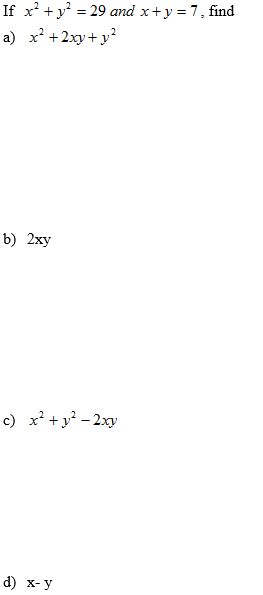e)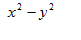10 marks

Back Top